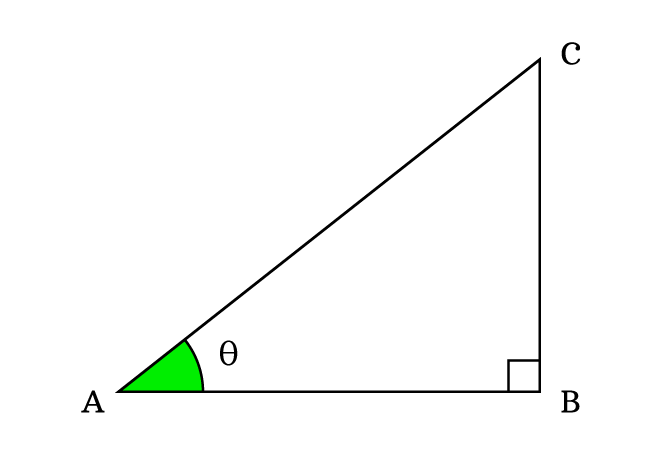# Proof for Cosine by Sine Quotient identity

According to the quotient of cosine by sine identity, the quotient of cosine by sine is equal to the cotangent.

$\dfrac{\cos{\theta}}{\sin{\theta}} \,=\, \cot{\theta}$

The trigonometric quotient identity is derived geometrically in mathematical form from a right triangle. Now, it’s your time to learn how to prove the quotient of cosine by sine identity from a geometric shape right triangle.

$\Delta BAC$ is a right angled triangle. In this triangle, $\overline{BC}$, $\overline{AB}$ and $\overline{AC}$ are opposite side, adjacent side and hypotenuse respectively, and the angle of this triangle is taken as theta.### Define Cosine and Sine functions

According to the definitions of the trigonometric functions, express the sine and cosine functions in ratio form at an angle theta.

$\sin{\theta} \,=\, \dfrac{BC}{AC}$

$\cos{\theta} \,=\, \dfrac{AB}{AC}$

### Divide the Cosine by Sine function

Now, divide the cosine function by the sine function for evaluating the quotient of them.

$\dfrac{\cos{\theta}}{\sin{\theta}} \,=\, \dfrac{\dfrac{AB}{AC}}{\dfrac{BC}{AC}}$

$\implies$ $\dfrac{\cos{\theta}}{\sin{\theta}}$ $\,=\,$ $\dfrac{AB}{AC} \times \dfrac{AC}{BC}$

$\implies$ $\dfrac{\cos{\theta}}{\sin{\theta}}$ $\,=\,$ $\dfrac{AB}{BC} \times \dfrac{AC}{AC}$

$\implies$ $\dfrac{\cos{\theta}}{\sin{\theta}}$ $\,=\,$ $\dfrac{AB}{BC} \times \require{cancel} \dfrac{\cancel{AC}}{\cancel{AC}}$

$\implies$ $\dfrac{\cos{\theta}}{\sin{\theta}}$ $\,=\,$ $\dfrac{AB}{BC} \times 1$

$\implies$ $\dfrac{\cos{\theta}}{\sin{\theta}}$ $\,=\,$ $\dfrac{AB}{BC}$

### Express the Ratio in Trigonometric function

We have derived in the above step that the ratio of cosine by sine is equal to the quotient of lengths of adjacent side ($AB$) by opposite side ($BC$).

$\dfrac{\cos{\theta}}{\sin{\theta}}$ $\,=\,$ $\dfrac{AB}{BC}$

According to the trigonometry, the quotient of $AB$ by $BC$ represents the cotangent. Here, the angle of right triangle is theta. Therefore, the cot function is written as $\cot{\theta}$.

$\,\,\,\therefore\,\,\,\,\,\,$ $\dfrac{\cos{\theta}}{\sin{\theta}}$ $\,=\,$ $\cot{\theta}$

Therefore, it is proved that the quotient of cosine function by sine function is equal to the cotangent function mathematically.

Latest Math Topics
Jun 26, 2023
Jun 23, 2023

Latest Math Problems
Jul 01, 2023
Jun 25, 2023
###### Math Questions

The math problems with solutions to learn how to solve a problem.

Learn solutions

Practice now

###### Math Videos

The math videos tutorials with visual graphics to learn every concept.

Watch now

###### Subscribe us

Get the latest math updates from the Math Doubts by subscribing us.